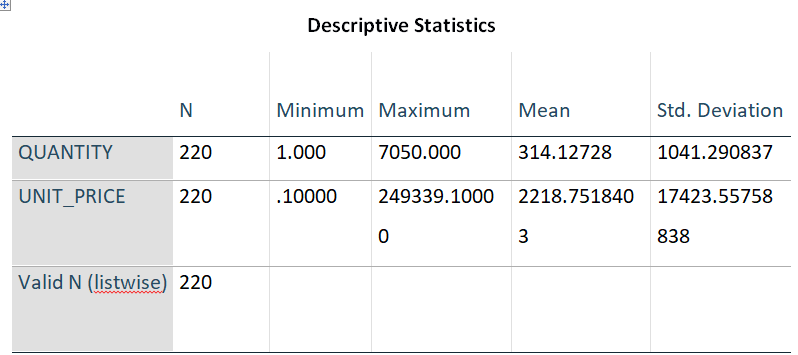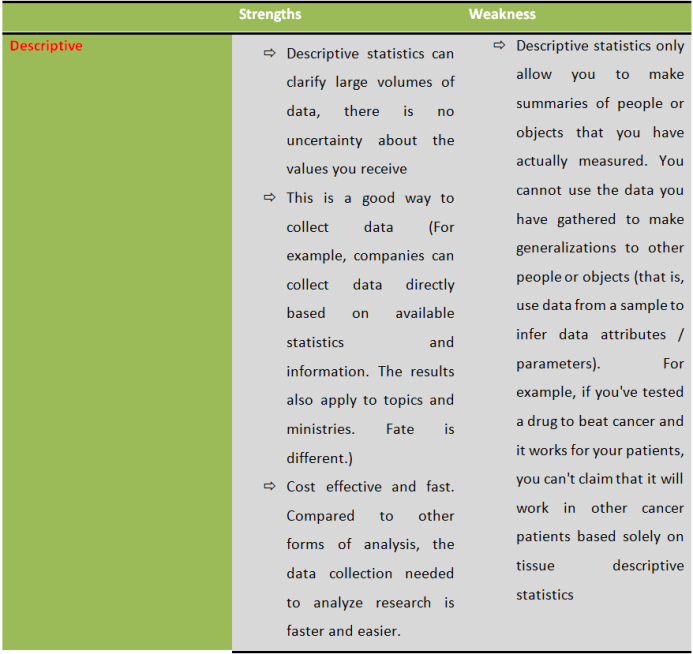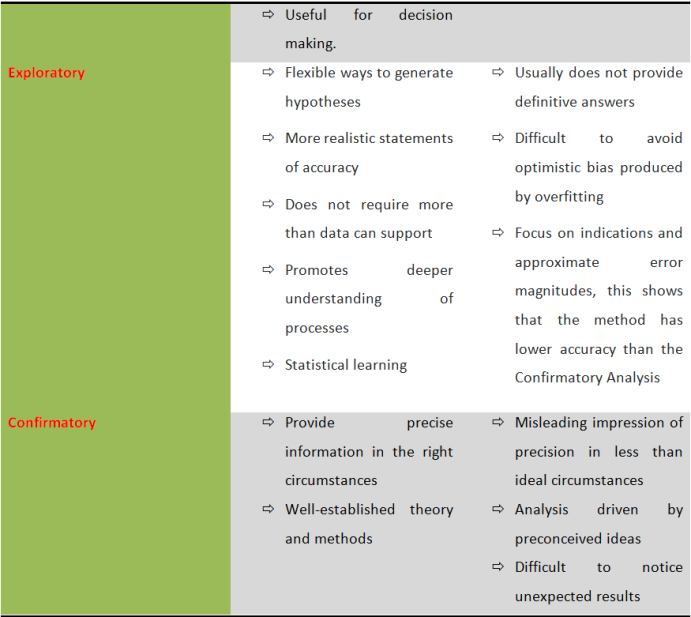# Statistics 1

## Evaluate data from a variety of sources using different methods of analysis.

1)  Interpreting data from a variety of sources using different methods of analysis:

###### ·       Descriptive:

Descriptive statistics are a short description of the data. In other words, this is an analytical method that summarizes a given data set. The summarized data may represent all collected data. Descriptive statistics include two measurement methods: measures of central tendency and measure of variability. Measures of central tendency have Mean, Median, Mode, Percentiles, Quartiles; while the Measures of variability include Range, Interquartile range, Variance, Standard deviation, Coefficient of Variation. In general, this method helps you to describe and understand the properties of a particular dataset by providing brief summaries of the sample and its parameters (Thao, 2019).Descriptive statistics are used to describe the basic features of data in a study. Descriptive statistics are often used in presenting a simple quantitative analysis of data. That is, in one study, too many variables are often measured and descriptive statistics will be given to break this amount of data into a simple form. In summary, descriptive statistics are used primarily to provide descriptions of the behavior of sample data, which helps us to simplify large amounts of data sensibly and greatly reduce data into a simpler summary (Diana, 2019).

###### ·       Exploratory

Exploration data analysis (EDA) is the first and important step in our data analysis process. It will help you understand the meaning of the data you have collected earlier. For this method of analysis, we have many ways to approach it. To approach with this method, you need to find out what data to create, set the questions you want to ask and how to organize them, as well as how to present and manipulate data. In general, this method helps you to draw important data (Blitz, 2017)

This is the method used to discover large data sets that will draw conclusions or predictions. In other words, it is used to answer questions, test business assumptions, create hypotheses for further analysis or to prepare data for modeling. The whole point of these practices is that solid data knowledge can help researchers get the answers they need or develop intuition to interpret results of future modeling (Blitz, 2017).

###### ·       Confirmatory

Confirmatory Data Analysis (CDA) is a data analysis process, in which you evaluate your evidence using traditional statistical tools such as significance, inference, and confidence. Analyzing validation data involves things like testing hypotheses, making high-precision estimates, regression analysis (estimating the relationship between variables) and variance analysis (assess the difference between actual and planned results). This will be the way you put your findings and reasoning into practice (Blitz, 2017).

The CDA allows people to assess the appropriateness between observed data and prioritize modeling based on concepts. The confirmatory data analysis is implemented in three directions: Firstly, apply the results obtained on a sample to the entire dataset; Secondly, proof of hypotheses (e.g. customers with an instant access savings account and a personal equity plan); Finally, checking cause-effect relations. In all three directions, we have a common process for it:

- Review the relevant theories or research papers that are relevant to it

-  Specify a model (for example: diagram, equations)

-  Determine model identification

-  Data collection

-  Conduct descriptive statistical analysis (e.g. scaling, data description)

-  Estimate the parameters in the model

-  Evaluate the data model you are researching

-  Present and interpret results (Mueller and Hancock, 2001).

2)  Demonstrate the strengths and weaknesses of every analysis method:Do Trung Huy

My name is Huy. I am studying at University of Greenwich in Vietnam. I live in 279/18 A1 Binh Tien, Distrist 6, Ho Chi Minh. Today, I want to find the online jobs and I think that Publish0x is trustworthy and suitable for me.